# Area + area of the shape - math problems

#### Number of problems found: 67

• The length 8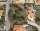The length of a rectangular field is x + 4 and its width is x + 2. what is the area of the field? Answer in polynomials expressions.
• The widthThe width of a rectangular garden is 4 m less than the length. If the area of a rectangular garden is 96 square meters, what is the dimension of the garden?
• The isoscelesThe isosceles trapezoid ABCD has bases of 18 cm and 12 cm. The angle at apex A is 60°. What is the circumference and content of the trapezoid?
• The area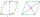The area of a rhombus is 143 m2. If the longer diagonal is 26 m, find the shorter diagonal in inches named d1.
• The conical roof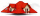The conical roof above the warehouse has a diameter of the lower part (base) d = 11.2 m and a height v = 3.3 m. How many rectangular steel plates with dimensions of 1.4 m and 0.9 m were needed for the production of this roof, if the seams and waste requir
• The triangleThe triangle has sides 5 cm long, 5 cm, 8 cm long. What is the area of the triangle?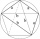The quadrilateral ABCD is composed of two right triangles ABD and BCD. For side lengths: |AD| = 3cm, | BC | = 12cm, | BD | = 5cm. How many square centimeters (area) does the quadrilateral ABCD have? The angles DAB and DBC are right.
• The collar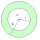The collar on the dress has the shape of an annulus 6 cm wide. The circumference of the inner circle is 31.4 cm. How much cm2 of fabric is needed to make one collar?
• The diamondThe diamond has an area S = 120 cm2, the ratio of the length of its diagonals is e: f = 5: 12. Find the lengths of the side and the height of this diamond.
• The kitchenThe kitchen has the shape of a square with a side of 5 m. We place linoleum on the floor, the price of which is 450 CZK per 1 m2. The glue costs 750 CZK. How much do we pay in total?
• The roofThe roof of the tower has the shape of a regular quadrangular pyramid, the base edge of which is 11 m long and the side wall of the animal with the base an angle of 57°. Calculate how much roofing we need to cover the entire roof, if we count on 15% waste
• The gardenThe garden has the shape of an isosceles trapezoid whose bases are 64 m and 24 m long, the height is 25 m. On what area of the garden is it possible to grow vegetables if a fifth of the area is occupied by a cottage, lawn and path?
• The resistanceWhat is the resistance of an aluminum wire 0.2 km long and 10 mm in diameter?
• The ABCDThe ABCD trapezoid has a base length of a = 120mm, c = 86mm and an area of S = 2575 mm2. Calculate the height of the trapezoid.
• The land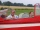The land, which has dimensions of 220 m and 308 m, the owner wants to divide into equally large square plots with the largest possible area. How long will one side of the plot be?
• The poolThe cube-shaped pool has 140 cubic meters of water. Determine the dimensions of the bottom if the depth of the water is 200 cm and one dimension of the base is 3 m greater than the other. What are the dimensions of the pool bottom?
• The square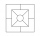Calculate the area and perimeter of a square with side a = 15 dm S =, O =
• The regularThe regular triangular prism has a base in the shape of an isosceles triangle with a base of 86 mm and 6.4 cm arms, the height of the prism is 24 cm. Calculate its volume.
• The funnelThe funnel has the shape of an equilateral cone. Calculate the content of the area wetted with water if you pour 3 liters of water into the funnel.
• The trench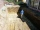Calculate how many cubic meters of soil needs to be removed from the excavation in the shape of an isosceles trapezoid, the top width is 3 meters, the lower width is 1.8 m, the depth of the excavation is 1 m, and the length is 20 m.

Do you have an exciting math question or word problem that you can't solve? Ask a question or post a math problem, and we can try to solve it.

We will send a solution to your e-mail address. Solved examples are also published here. Please enter the e-mail correctly and check whether you don't have a full mailbox.

Area - math problems. Examples of area of plane shapes.